HomeBackgroundResourceTechnologyEnvironmentMethodologyCase StudyConclusionsReferencesTeam ESRU Energy Systems Research Unit###### MARINE CURRENT RESOURCE AND TECHNOLOGY METHODOLOGY# Technology: Turbine Theory

Actuator Disk Theory    Rotational InductionHAMCT Glauert's Blade Element Momentum Theory (HAMCT)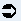VAMCT The Double-Multiple Streamtube Model (VAMCT)## Froude's Momentum Theory for an Actuator Disk

Froude’s actuator disk theory provides a simple, 1-dimensional approach to the problem of rotor modelling. Armed with the following assumptions consider an ideal rotor as shown below.

1. 1-Dimensional analysis and disk is essentially a discontinuity moving through the fluid
2. Infinitesimally thin disk of area A which offers no resistance to fluid passing through it as frictional forces are negligible compared with momentum flux and pressure changes (hence can make assumption 5)
4. Far-field is at free-stream pressure but far up and downstream
5. Inviscid (thus irrotational), incompressible and isentropic flow

Since the disk acts as a drag device, and by assumption 1, the source of drag must be a pressure difference across the disk and this drag manifests itself as thrust loading along the axis normal to the disk. Rewriting N2 in terms of momentum:Eqn 1

A relationship between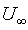,u1,u2, Thrust (slowing the fluid) and Power may be derived by consideration of the assumptions an N2. Noting that assumption 1 states the disk is a discontinuity and that the flow can be considered stationary, Bernoulli’s equation and constant are not valid and may not be applied across the disk, but may be applied from the far field to the disk in either direction.
Placing a control volume around the disk extending far ahead to the free-stream and considering that the disk serves to slow down the flow, thus allowing mass conservation to define streamlines (as the flow slows, conservation dictated the area increases):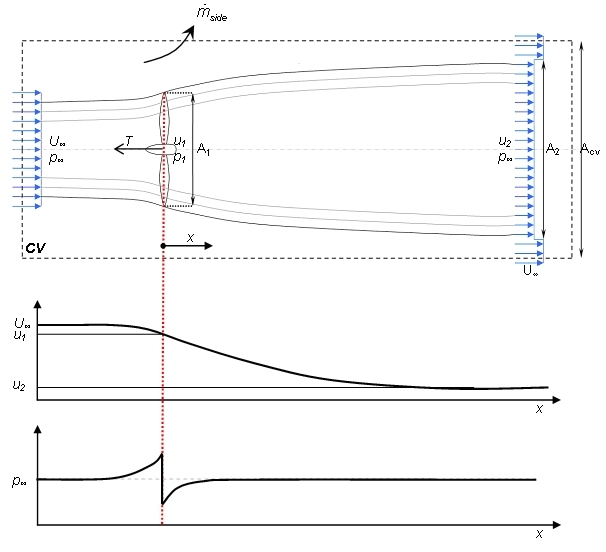Applying Bernoulli in valid regions: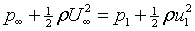Eqn 2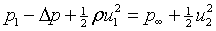Eqn 3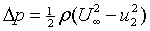Eqn 4

Applying the axial momentum equation to a cylindrical control volume (CV) made by rotation of above control volume about the x-axis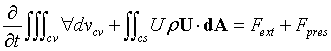Eqn 5

dA is a unit vector normal to the integral element (of unit area) of control volume in question;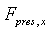is the axial component of pressure forces acting on the c.v. Simplifying this equation by assuming stationary flow (ie. a moving disk) and noting that pressure forces on the ends of the c.v. are equal (at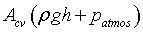) thusand we have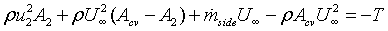Eqn 6

Conservation of mass yields the following relationships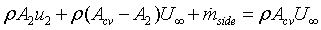Eqn 7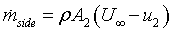Eqn 8Eqn 9

Which when combined with Eqn 6 gives the following relationship, as anticipated by (and in the expected form of) Eqn 1.Eqn 10 & by eqn 4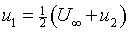Eqn 11

Thus the velocity at the actuator disk is the mean of the freestream and far wake velocities.
Redefining the control volume to be co-incident with steamlines around the disk, in other words ensuring no mass transfer out of the c.v. except at the ends (as the fluid flows along streamlines and cannot cross them). Thus recasting the axial momentum equations as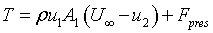Eqn 12

And noting that the physics of the situation are the same, no matter which c.v. is used, it is possible to declare the net pressure force on the c.v. walls that follow streamlines must be zero.
By assumption 4 and referring to the new control volume, power is equal to the rate of work, which is itself equal to the rate of momentum transfer through the c.v and is given byEqn 13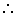Eqn 14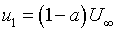Eqn 15

defining an axial induction factor a and by combining 15 & 11well known resultEqn 16 thusEqn 17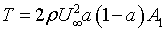Eqn 18

Defining CP as power non dimensionalised by available power through disk area, and similarly defining CT forthrust,Eqn 19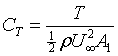Eqn 20 and by 17, 18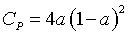Eqn 21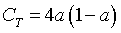Eqn 22

Differentiating CP w.r.t. a yieldsEqn 23

Which when equated to zero yields a theoretical maximum value of CP (16/27 at a = 1/3) for an actuator disk, known as the Lanchester-Betz limit after Frederick Lanchester and Albert Betz, of whom one worked with Ludwig Prandtl and the other was an all round genius (and a Brit).

This simple momentum theory is only valid for a certain range of values of a, above which physically unrealisable results are obtained as there occurs flow reversal in the wake and eventually the vortex ring state, so dreaded by helicopter pilots.

From here the derivation of the vertical axis turbine model can proceed and with rotational adjustments the horizontal axis model derivation may continue.

## Rotational considerations

Assumption 5 states that the ideal rotor considered experiences irrotational flow, which is intuitively incorrect unless there is some sort of pre-rotator/stator arrangement to ensure an irrotational wake, or a contra-rotating coaxial turbine. While these are both feasible options, as assumptions they remove generality from the model, and as such the model is developed assuming some amount of rotation in the wake.
The Euler turbomachinery equation applied to an annular control volume of infinitesimal dimensions is written in cylindrical (r,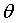,x) coordinates asEqn 24

Where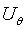indicates azimuthal component of absolute velocity U immediately after the rotor and u1 is axial velocity through the rotor. The velocity triangles in the figure below show the relation between tangential velocity (due to rotation), absolute, normal and resultant velocities for as section of the rotor. By equation 1, for a given power and freestream velocity, rotational velocity componentpost rotor degreases with an increase in rotor angular velocity, Omega. In a similar manner as in the preceeding section, a rotational or tangential induction factor a’ may be defined where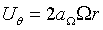Eqn 25Eqn 26 and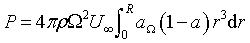Eqn 27 and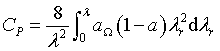Eqn 28; with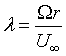Eqn 29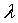is the tip speed ratio (TSR) and (31) is the local tip speed ratio at radius r.

By consideration of the following diagram a relationship between axial and tangential induction factors may be obtained (blade mortion is downwards):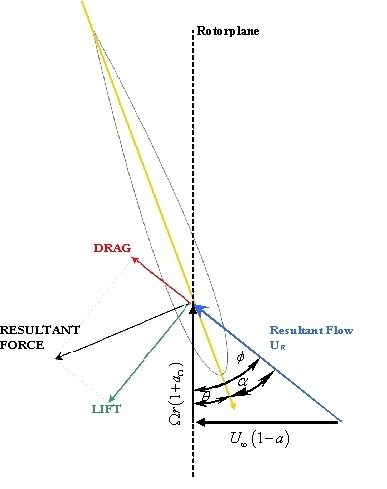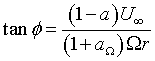Eqn 30

Where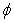is the angle between the aerofoil zero lift line and the resultant velocity.

## References & Bibliography

 1 HANSEN, M.O.L., Aerodynamics of Wind Turbines : Rotors, Loads and Structure. 2001, London: James & James (Science Publishers) Ltd. pp. 152. ISBN 1902916069. 2 BURTON, T., et al., Wind Energy Handbook. 2001, Chichester, New York: John Wiley and Sons Ltd. pp. 624. ISBN 0471489972. 3 CHANEY, K. and EGGERS, A.J., Expanding Wake Induction Effects on Thrust Distribution on a Rotor Disc Wind Energy, 2001(5): p. 213-226.

 Glauert's Blade Element Momentum Theory (HAMCT)The Double-Multiple Streamtube Model (VAMCT)Go back to Contents